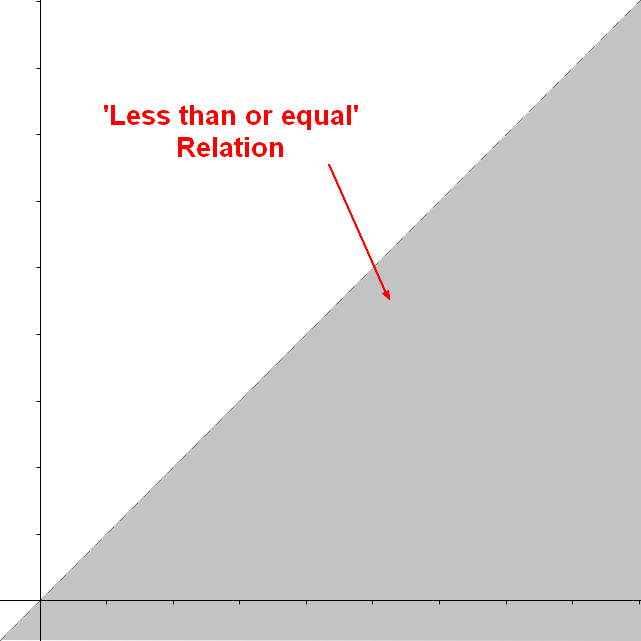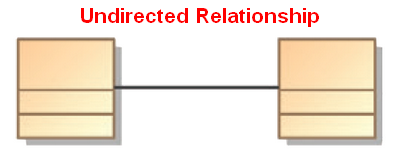# Logical Data Modeling - Equivalence Relationship (Equality)

The equivalence relation is a relationship between two entities.

The equivalence relation is also known as:

• is equal to.
• equality
• is parallel to (for affine spaces)
• is in bijection with - bijection
• isomorphy

## Properties

An equivalence relation is a binary relation that is:

Recommended PagesCollection - Sequence (Ordered)

A sequence is an abstract collection: of non-unique element where order matters - ie (A,B) is not equal to (B,A) non-unique means that it allows duplicate member (the same element/value may occur...Data Modeling - Canonical Form

A canonical form is a unique representation such that every object can be uniquely identified in the global scope object (entity)normal form In computer science, the equality of two objects can easily...
Javascript - Set

The Set interface represents a set data type and works only with primitive type as there is no way to define an object equality function. object Jsdoc Declaration Add/Delete Size Contains...Language - Equality

The Equality operators model the Equivalence relation: equality is parallel to (for affine spaces) is in bijection with isomorphy The Equality operators are a subset of the comparison operator....Logical Data Modeling - Binary Relation

A binary relation is a relationship between two elements that is implemented via a binary function. Binary relations are used in many branches of mathematics to model concepts like: order relation...Logical Data Modeling - Duplicate

A duplicate happens when in a set two elements have an equivalence relationship that returns true. In the set {1,1,2,3}, 1 is a duplicate The set {1,2,3} has no duplicate relational operators...Logical Data Modeling - Reflexive relationship property

reflexive is a relationship property that indicates: that the relationship relates every element to itself. in other word, that the relation set representing the relationship contains every tuple:...Logical Data Modeling - Symmetric relationship (bi-directional)

A symmetric relation is a type of binary relation that happens when for all a and b in X if a is related to b, then b is related to a In mathematical notation, the relation f between x and y is...Logical Data Modeling - Transitive Relationship Property

transitive is a relationship property that tells that the relationship follows the following rule: when the relation relates a to b and b to c, then the relation relates a to c. In mathematical notation,...Ordinal Data - Comparison Operator (Order, Equality)

A comparison operator is a class of operators that model: a order or a equivalence relationship A comparison operator test the order relationship between two operands and returns a Boolean. The...